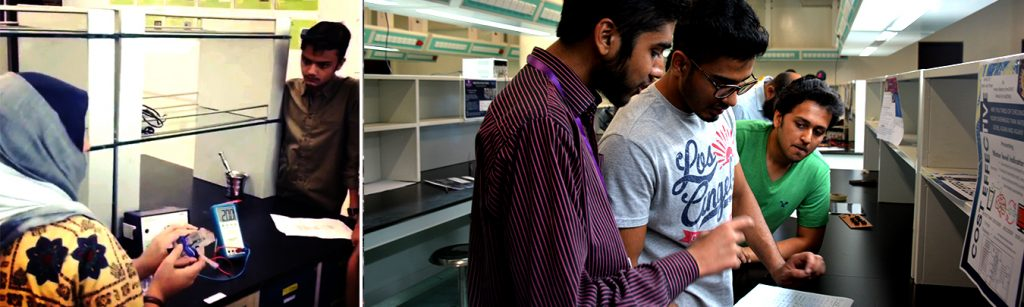Habib Response - Covid-19The Advanced Physics Lab is designed to provide students the opportunity to demonstrate some of the basic ideas in Solid State Physics, Electromagnetism, Materials and many other fields of Modern Physics. The Advanced Physics Lab has the following experimental setups:

Measurement of Planck’s constant
In this experiment, we use a normal incandescent bulb to determine the fundamental Planck’s constant. This is done by observing the change in light intensity as a function of changing voltage across the incandescent bulb.
Measurement of band gap of germanium crystal
This apparatus measures the band gap of germanium crystals by observing the change in resistance of the crystal as a function of changing temperature of the germanium strip.  The resultant data is plotted and fitted to determine the band gap of germanium.
Harmonic electricity generator
A pendulum made up of a neodymium magnet is made to oscillate between two sets of coils with 250 turns. The oscillating magnet causes magnetic flux through to coil to oscillate at the frequency of the pendulum. The frequency of the resultant AC current in the coil is measured and compared with the frequency of the oscillator.
Determining excitation potential of Ne between 2s to 3p states using a Frank-Hertz setup
The setup mimics the famous Frank-Hertz experiment which confirmed the existence of quantized energy level in atoms. In this experiment we determine the energy level of Neon gas. We also use mathematical models to calculate the energy distribution and the number of electrons emitted by the filament.
Determination diffraction grating constant and light source identification
The experiment demonstrates the diffraction of light in various gas lamps. The light is incident on a diffraction grating of various grating values and the resultant diffraction pattern of light is observed for various gas lamps.
Determination of density and mobility of charge carriers in dopped germanium
Hall effect is the phenomena of collection of charges along the sides of a flat conductor under the influence of a perpendicular magnetic field. This experiment is designed to study the Hall effect in germanium crystal. The charge carrier density and hall coefficient for germanium is also determined. The same equipment is used to demonstrate the use of hall effect in determining the unknown magnetic field.
Characteristics of solar cell and the effect of temperature on its characteristics
Voltage load characteristics of a solar cell is an important parameter in the operation of solar cell panel. In this experiment these characteristics of the given solar cell are studied for the given arrangement of solar panels.
Magnetic moment of a current loop and its interaction in a magnetic field
A sensitive torsion balance is used to determine magnetic moment of a 100 turn copper coil. The aim of the experiment is to determine the relationship between magnetic field strength and deflection of the coil in a magnetic field.
Using Ampere’s law to determine the magnetic field of a solenoid
A solenoid is a straight wire which is wound into a number of turns, like a spring. When current is made to pass through the coil which generates a constant, unidirectional magnetic field in the interior of the coil. The strength of the magnetic field depends on the length of the solenoid and magnitude of the current. In this experiment the model of magnetic field strength derived from Ampere’s law is verified.
Determination of gyromagnetic ratio of a proton
The experiment is designed to introduce you to the concept of NMR and the basic Quantum Mechanical theory behind it. You will also measure the gyromagnetic ratio of the proton.
Determination of charge-to-mass ratio (e/m) using a magic eye
e/m ratio of a particle is the ratio of its charge to its mass. In this experiment this quantity is determined for the electron using a cathode tube triode called a magic eye. The device is called a magic eye for its greenish hue of electron which looks like an eye.
Determination of refractive index of air using Mach-Zehnder interferometer
Mach-Zehnder interferometer introduces the basic working concepts of interferometer. In addition, it also used to find out the refractive index of air.
Studying the effects of magnetic breaking due to Eddy currents in conductors
Eddy currents are the current produced in the conductor as a result of alternating magnetic field. The experiment demonstrates the basic idea of magnetic breaking.